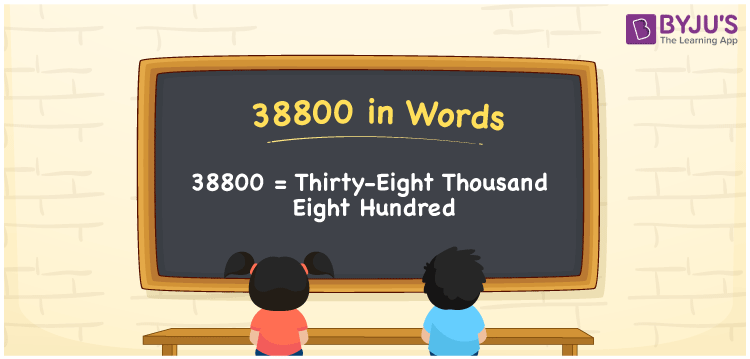# 38800 in Words

38800 in words is represented by Thirty-Eight Thousand Eight Hundred. For example, if you paid Rs. 38800 for purchasing an iPad, you can write, “I paid Rupees Thirty-Eight Thousand Eight Hundred for purchasing an iPad”. Using a place value of a given number helps us to produce a number name with ease. 38800 is a cardinal number as it shows some quantity or value. This article describes how to write and spell the number 38800 in English with the help of simple tricks.

 38800 in Words Thirty-Eight Thousand Eight Hundred Thirty-Eight Thousand Eight Hundred in numerical form 38800

## 38800 in English Words

Generally, we write the numbers in words using the letters present in the English alphabet. Hence, in English, we can read the number 38800 as Thirty-Eight Thousand Eight Hundred.## How to Write 38800 in Words?

The place value chart for the number 38800 is provided below along with the expanded form.

 Ten-Thousands Thousands Hundreds Tens Ones 3 8 8 0 0

Hence, we can write the expanded form as:

3 x 10000 + 8 x 1000 + 8 x 100 + 0 x 10 + 0 x 1

= 30000 + 8000 + 800 + 0 + 0

= 30000 + 8000 + 800

= 38800

= Thirty-Eight Thousand Eight Hundred

Therefore, 38800 in words is written as Thirty-Eight Thousand Eight Hundred

Interesting way of writing 38800 in words

3 = Three

38 = Thirty-Eight

388 = Three Hundred and Eighty-Eight

3880 = Three Thousand Eight Hundred Eighty

38800 = Thirty-Eight Thousand Eight Hundred

Thus, the word form of the number 38800 is Thirty-Eight Thousand Eight Hundred

38800 is a natural number that is the successor of 38799 and the predecessor of 38801

• 38800 in words – Thirty-Eight Thousand Eight Hundred
• Is 38800 an odd number? – No
• Is 38800 an even number? – Yes
• Is 38800 a perfect square number? – No
• Is 38800 a perfect cube number? – No
• Is 38800 a prime number? – No
• Is 38800 a composite number? – Yes

## Frequently Asked Questions on 38800 in Words

Q1

### Write 38800 in words.

38800 in words is written as Thirty-Eight Thousand Eight Hundred.
Q2

### Simplify 30000 + 8800, and express in words.

Simplifying 30000 + 8800, we get 38800. The number 38800 in words is Thirty-Eight Thousand Eight Hundred.
Q3

### 38800 is a composite number. True or False.

True, the number 38800 is a composite number.